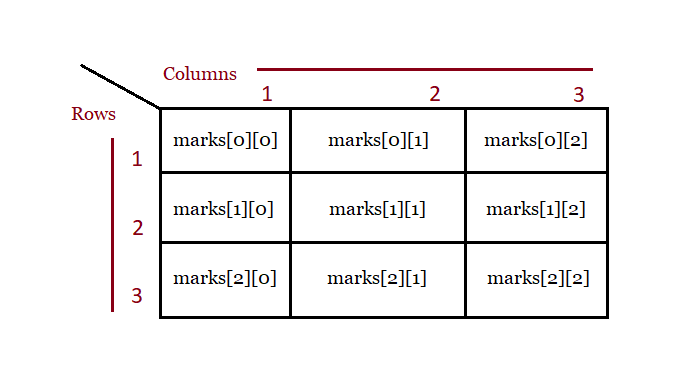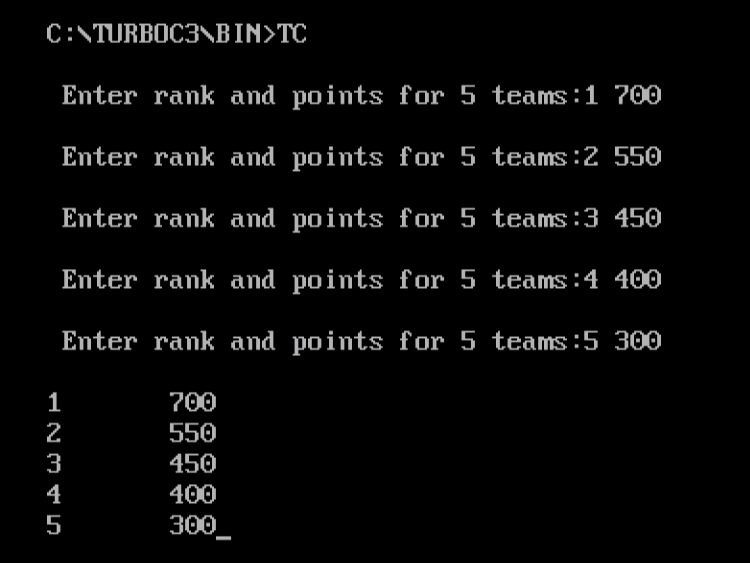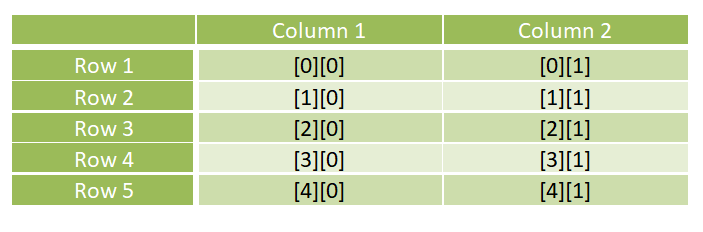# Two Dimensional Arrays in C++

## 12 Jan Two Dimensional Arrays in C++

In the previous lesson, we learn how to work with Arrays in C++. An array can have two or more dimensions. Here we will discuss about two-dimensional arrays. A two dimensional array as the name suggests has two dimensions, not what we saw in one-dimensional arrays.

Before the example, let us see the syntax,

Considering the above syntax, let us see an example, with 3 rows and 3 columns in a two-dimensional array,

Let us now see how rows and columns work in a two-dimensional array,Now, let us see a complete example to learn more about two-dimensional arrays.

### Example

Let us see a program where we will print the rank and points of 5 cricketers. Here, you can see an array with rows and columns i.e. two-dimensional.

We’re displaying the rank and points for 5 teams, but before that you need to input the values. The cin in C++ is used to input values and cout to get the output,

Here, cric would mean 1st row and 1st column, cric is 1st row and 2nd column, etc

The following is the output,Here, you saw the following two dimensional arrays,In this lesson we learned how to work with Multi-Dimensional array in C++.[email protected]

We work to create free tutorials for all.